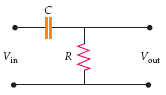# Phase constant for high pass filter

## Homework StatementIf the input voltage is given by Vin = Vin peak cos ωt, then the output voltage is
Vout = VH*cos(ωt - δ) where VH = Vin peak/(1 + (ωRC)^(−2)) (Assume that the output is connected to a load that draws only an insignificant amount of current.)

Find an expression for the phase constant δ in terms of ω, R and C.

## The Attempt at a Solution

My teacher specifically told us to solve this problem without using phasors, but the only examples in the book of how to solve this kind of problem use phasors. I know that the answer is arctan(-1/(ωRC)), but I have no idea how to derive that!

collinsmark
Homework Helper
Gold Member
Hello dstdnt,

Welcome to physics forums!

The way I would do it is, in the diagram, replace the label "C" with the capacitor's impedance. In other words, replace "C" with "1/(jωC)". Then the circuit is just a simple voltage divider. Solve for Vout in terms of Vin.

The phase is arctan([imaginary part]/[real part]). That's almost the same thing as δ. [Notice the problem statement defined Vout = VH*cos(ωt - δ), where there is a minus sign attached to δ. You'll have to take that into account]